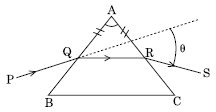NEET Physics Ray Optics and Optical Instruments Questions Solved

A ray PQ incident on the refracting face BA is refracted in the prism BAC as shown in the figure and emerges from the other refracting face AC as RS such that AQ = AR. If the angle of prism A = $60°$ and refractive index of material of prism is $\sqrt{3}$, calculate angle $\mathrm{\theta }$.Explanation is a part of a Paid Course. To view Explanation Please buy the course.

Difficulty Level: